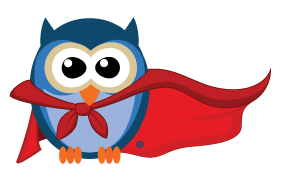• SKU: BBT-GLOW

\$99.00

# Glow

• Number of Students: 1-2 per Glow
• Number of Lessons: 9+ Practice Modes, 35+ levels
• Contact Hours: N/A
• Additional Resources: Glow Teacher Guide

Highlights:

GLOW: A TOOL FOR STUDENTS TO SEE MATH IN A NEW LIGHT!

• 9+ practice modes across apps
• 35+ levels to build skills
• 1 tablet, Chromebook, or Mac required
Glow is part LED display, part etch-a-sketch, and all about making math make sense! Students turn dials and press buttons that control the lights and, in turn, illustrate operations, write math equations, and model fractions. The hands-on aspect allows students to visualize math concepts in a new, concrete way.

Glow Apps
• GlowGrid - Students model addition, subtraction, multiplication, and division with lights
• GlowPix - Students write math equations to create pictures
• Fractions - Students model fractions

Teacher Guide
Teachers love Glow for self-checking, differentiation, and reporting. Whether using Glow to introduce a standard, revisit a skill, or offer remediation, the Teacher Guide offers helpful sample introduction scripts, student prompts, and discussion questions.

The Owlet Math Tools Collection

Two hands-on manipulatives, Glow and Cube, combine with digital apps to make abstract math concrete for grades K-5. “Aha!” moments result from peer-to-peer sharing, safe space to explore, and immediate feedback.

Based in research, driven by joy

• The Owlet Math Tools collection is the result of a 3 year design and research study supported by the National Science Foundation and enhanced by real math teachers in classrooms across the United States.

No-distractions math manipulatives for K-5

• Let students explore math in a safe, ad-free digital app. With Glow and Cube, engagement comes naturally - no games required. Align to learning goals while practicing math that actually looks like math.

Curriculum or Lesson Topics:

GlowGrid App:

Categories:

• Subtraction
• Multiplication
• Division
• Division with Remainders

Activity Modes:

• Explore: Turn dials to see how the equation cahnges in the app
• Make: Make a target number (only for Addition 1 & 2, Subtraction 1 & 2, and Multiplication)
• Solve: Solve arithmetic problems
• Find: Solve missing number problems

GlowPix App:

Levels:

• Level 1: Add and subtract 1 and 10 to move from one number to another
• Level 2: Add and subtract to move from one number to another
• Level 3: Add and subtract to select numbers from 1-120
• Level 4: Use all four operations to select numbers from 1-144
• Level 5: Use parentheses to create more complex equations

Fractions App:

Categories:

• Foundations: Exploring unit fractions and dividing a whole into equal parts
• Intro: Begin to work with fractions and mixed numbers
• Equivalence: Equivalent fractions

Activity Modes:

• Explore: model fractions
• Make: Make fractions from prompts
• Build: Make a fraction, then find other fractions that are equal to it (only in Intro)
• Compare: Make two fractions and then compare them (only in Intro)

What’s Included:

• 1 Glow LED board
• 1 USB charging cable
• 5 overlays for the LED board